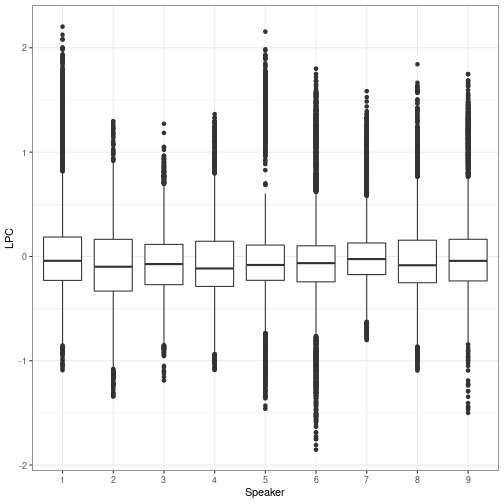# Classification with Reservoir Computing

Reservoir Computing (RC) is well suited to both regression and classification tasks. In the following notebook, you will experiment with a simple example of classification task.

library(reservoirnet)
library(ggplot2)

# Classification - The Japanese vowel dataset

The Japanese vowel dataset is composed of 640 utterances of the Japanese vowel , from 9 different male speakers. The goal of this task is to assign to each utterance the label of its speaker. Dataset is split between a 270 utterances training set and a 340 utterances testing set.

Each spoken utterance is a timeseries of 7~29 timesteps. Each timestep of signal is a 12 dimensional vector representing Linear Prediction Coefficient (LPC), which encode the audio signal into the cepstral domain (a variant of the frequency domain).

References M. Kudo, J. Toyama and M. Shimbo. (1999). “Multidimensional Curve Classification Using Passing-Through Regions”. Pattern Recognition Letters, Vol. 20, No. 11–13, pages 1103–1111.

https://archive.ics.uci.edu/ml/machine-learning-databases/JapaneseVowels-mld/

japanese_vowels <- reservoirnet::generate_data(
dataset = "japanese_vowels")$japanese_vowels X_train <- japanese_vowels$X_train
Y_train <- japanese_vowels$Y_train X_test <- japanese_vowels$X_test
Y_test <- japanese_vowels$Y_test # Echo state network setup First we will define a reservoir network : sample_per_speaker <- 30 n_speaker <- 9 X_train_per_speaker <- list() for (i in 0:8) { X_speaker <- X_train[((i*sample_per_speaker)+1):((i+1)*sample_per_speaker)] X_train_per_speaker[[i+1]]<-(as.numeric(unlist(sapply(X_speaker, t)))) } d <- data.frame(LPC = unlist(X_train_per_speaker), Speaker = factor(rep(1:9,times = sapply(X_train_per_speaker,length)))) ggplot(d,aes(x = Speaker, y = LPC)) + geom_boxplot()+theme_bw()plot of chunk boxplotvowel ##Transduction (sequence-to-sequence model) As ReservoirPy Nodes are built to work on sequences, the simplest setup to solve this task is sequence-to-sequence encoding, also called transduction. A model is trained on encoding each vector of input sequence into a new vector in the output space. Thus, a sequence of audio yields a sequence of label, one label per timestep. japanese_vowels <- reservoirnet::generate_data( dataset = "japanese_vowels", repeat_targets=TRUE)$japanese_vowels

X_train <- japanese_vowels$X_train Y_train <- japanese_vowels$Y_train
X_test <- japanese_vowels$X_test Y_test <- japanese_vowels$Y_test

## Train a simple Echo State Network to solve this task:

source <- createNode("Input")
reservoir <- createNode("Reservoir",units = 500,lr=0.1, sr=0.9)

#[source >> reservoir, source] >> readout
model <- list(source %>>% reservoir, source) %>>% readout

## Fit the model

model_fit <- reservoirnet::reservoirR_fit(node = model,
X = X_train,
Y = Y_train,
stateful = FALSE,
warmup = 2)

Y_pred <- reservoirnet::predict_seq(node = model_fit$fit, X = X_test, stateful = FALSE) Get the scores: There are 9 speakers, hence the output space is 9-dimensional. The speaker label is the index of the output neuron with maximum activation. accuracy <- function(pred, truth){ mean(pred == truth) } Y_pred_class <- sapply(Y_pred, FUN = function(x) apply(as.matrix(x),1,which.max)) Y_test_class <- sapply(Y_test, FUN = function(x) apply(as.matrix(x),1,which.max)) score <- accuracy(array(unlist(Y_pred_class)), array(unlist(Y_test_class))) print(paste0("Accuracy: ", round(score * 100,3) ,"%")) ##  "Accuracy: 91.296%" # Classification (sequence-to-vector model) We can create a more elaborated model where inference is performed only once on the whole input sequence. Indeed, we only need to assign one label to each input sequence. This new setup is known as a sequence-to-vector model, and this is usually the type of model we refer to when talking about classification of sequencial patterns. japanese_vowels <- reservoirnet::generate_data( dataset = "japanese_vowels")$japanese_vowels

X_train <- japanese_vowels$X_train Y_train <- japanese_vowels$Y_train
X_test <- japanese_vowels$X_test Y_test <- japanese_vowels$Y_test
source <- reservoirnet::createNode("Input")
reservoir <- reservoirnet::createNode("Reservoir", units = 500, lr=0.1, sr=0.9)

model <- source %>>% reservoir %>>% readout

We need to modify the training loop by hand a bit to perform this task:

first, we compute all reservoir states over the input sequence using the reservoir.run method. then, we gather in a list only the last vector of the states sequence.

states_train = list()
k <- 1
for (x in X_train) {
states <- reservoirnet::predict_seq(node = reservoir, X = x, reset=TRUE)
states_train[[k]] <- t(as.matrix(states[nrow(states),]))
k <- k+1
}

We can now train the readout only on the last state vectors. Here, Y_train is an array storing a single label for each utterance.

res <- reservoirnet::reservoirR_fit(readout,X = states_train, Y = Y_train)
summary(res)
## Parametrs using to fit:
##  warmup: 0 ; stateful: FALSE ; reset: FALSE
## results of fitting:
## 'Ridge-5': Ridge(ridge=1e-06, input_bias=True, in=500, out=9)

We also modify the inference code using the same method as above:

Y_pred <- list()
k <- 1
for (x in X_test) {
states <- reservoirnet::predict_seq(node = reservoir, X = x, reset=TRUE)
y <- reservoirnet::predict_seq(node = readout, X = as.array(states[nrow(states),]))
Y_pred[[k]] <- y
k <- k+1
}
Y_pred_class <- sapply(Y_pred, FUN = function(x) apply(as.matrix(x),1,which.max))
Y_test_class <- sapply(Y_test, FUN = function(x) apply(as.matrix(x),1,which.max))

score <- accuracy(pred = Y_test_class,
truth = Y_pred_class)

print(paste0("Accuracy: ", round(score * 100,3) ,"%"))
##  "Accuracy: 88.108%"